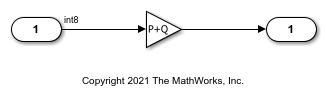# Remove Code from Tunable Parameter Expressions That Saturate Against Integer Overflow

Optimize generated code by removing code that protects parameter expressions from integer overflow for integers and fixed-point data. If you are sure that you will not tune the terms of tunable parameter expressions to cause them to evaluate to a value that is below or above the representable value of a data type, enable this optimization.This optimization increases execution speed and results in smaller code that reduces ROM consumption.

Risks

When you select the `EfficientTunableParamExpr` parameter, and you tune the terms of the expression so that it encounters an integer overflow, the result will wrap around in the generated code. This behavior can cause the application to behave unexpectedly at run-time.

NOTE: If you enable this optimization, it is possible that simulation results and results from generated code are not in bit-for-bit agreement. This example requires Embedded Coder®.

### Example Model

In the model matlab:mEfficientParamExpr, an input signal of type `int8` feeds into a Gain block.

```model = 'mEfficientParamExpr'; open_system(model); ```### Generate Code

In your system temporary folder, create another temporary folder for the build and inspection process.

```currentDir = pwd; [~,cgDir] = rtwdemodir(); ```

Build the model.

```set_param(model, 'EfficientTunableParamExpr', 'off'); evalc('slbuild(model)'); ```

View the generated code without the optimization. Here is a portion of `mEfficientParamExpr.c`.

```cfile = fullfile(cgDir,'mEfficientParamExpr_ert_rtw','mEfficientParamExpr.c'); rtwdemodbtype(cfile,'/* Model step function ','/* Model initialize function',1, 1); ```
```/* Model step function */ void mEfficientParamExpr_step(void) { int32_T tmp; /* Gain: '<Root>/Gain' */ tmp = P + Q; if (tmp > 127) { tmp = 127; } else if (tmp < -128) { tmp = -128; } /* Outport: '<Root>/Out1' incorporates: * Gain: '<Root>/Gain' * Inport: '<Root>/In1' */ rtY.Out1 = (int8_T)(tmp * rtU.In1); } ```

### Enable Optimization

1. Open the Configuration Parameters dialog box.

2. On the Optimization pane, select Remove code from tunable parameter expressions that saturates out-of-range values.

Alternatively, use the command-line API to enable the optimization:

```set_param(model, 'EfficientTunableParamExpr', 'on'); ```

### Generate Code with Optimization

The optimized code does not contain code that checks the bounds of the representable value of a data type.

Build the model.

```evalc('slbuild(model)'); ```

The following is a portion of `mEfficientParamExpr.c`. The code that protects against expression overflow is not in the generated code.

```rtwdemodbtype(cfile,'/* Model step function ','/* Model initialize function',1, 1); ```
```/* Model step function */ void mEfficientParamExpr_step(void) { /* Outport: '<Root>/Out1' incorporates: * Gain: '<Root>/Gain' * Inport: '<Root>/In1' */ rtY.Out1 = (int8_T)((int8_T)(P + Q) * rtU.In1); } ```

Close the model and code generation report.

```bdclose(model) rtwdemoclean; cd(currentDir) ```